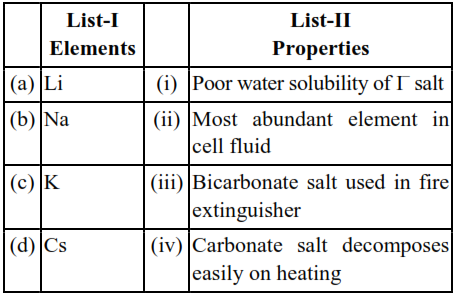# Match List I with List II :Question:

Match List I with List II :Choose the correct answer from the options given below:

1. (a)-(iv), (b)-(iii), (c)-(ii), (d)-(i)

2. (a)-(i), (b)-(iii), (c)-(ii), (d)-(iv)

3. (a)-(iv), (b)-(ii), (c)-(iii), (d)-(i)

4. $(\mathrm{a})-(\mathrm{i}),(\mathrm{b})-(\mathrm{ii}),(\mathrm{c})-(\mathrm{iii}),(\mathrm{d})-(\mathrm{iv})$

Correct Option: 1

Solution:

(a) $\mathrm{C}_{\mathrm{S}} \mathrm{I}$ salt is poor water soluble due to it's low hydration energy

(b) $\mathrm{NaHCO}_{3}$ is used in fire extinguisher

(c) $\mathrm{K}$ is most abundant element in cell fluid

(d) $\mathrm{Li}_{2} \mathrm{CO}_{3}$ decomposes easily due to high covalent character caused by small size $\mathrm{Li}^{+}$cation.